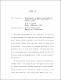## Uniqueness for continuous superresolution by means of Choquet theory and geometric measure theory

 dc.contributor.advisor Benedetto, John J en_US dc.contributor.author Cinoman, Ryan M en_US dc.contributor.department Mathematics en_US dc.contributor.publisher Digital Repository at the University of Maryland en_US dc.contributor.publisher University of Maryland (College Park, Md.) en_US dc.date.accessioned 2021-07-14T05:33:32Z dc.date.available 2021-07-14T05:33:32Z dc.date.issued 2021 en_US dc.description.abstract The problem of superresolution is to recover an element of a vector space from data much smaller than the dimension of the space, using a prior assumption of sparsity. A famous example is compressive sensing, where the elements are images with a large finite resolution. On the other hand, we focus on a continuous form of superresolution. Given a measure $\mu$ on a continuous domain such as the two dimensional torus, can we recover $\mu$ from knowledge of only a finite number of its Fourier coefficients using a total variation minimization method? We will see that the answer depends on certain properties of $\mu$. Namely, a necessary condition is that $\mu$ be discrete.We use methods from geometric analysis to investigate the continuous superresolution problem. Tools from measure theory relate properties of the support of a measure, such as Hausdorff dimension, to properties of its Fourier transform. We also use measure theory to investigate the possibility of alternatives to total variation that may allow us to recover surface measures defined on space curves. There is a theorem of Choquet concerning representations of points in convex sets as sums of their extreme points. As it turns out, we can apply this to the superresolution problem because the extreme points of the set of measures with total variation $1$ are precisely the set of delta measures. We consider superresolution as a convex optimization problem, where the goal is to find representations of the initial data as sums of delta measures. Choquet theory provides tools to investigate the previously unresolved problem of uniqueness. We use this to give a novel sufficient condition for a measure to be uniquely superresolved, given data on a known finite set of frequencies. en_US dc.identifier https://doi.org/10.13016/g9eu-t5fr dc.identifier.uri http://hdl.handle.net/1903/27443 dc.language.iso en en_US dc.subject.pqcontrolled Mathematics en_US dc.subject.pquncontrolled Fourier Analysis en_US dc.subject.pquncontrolled Harmonic Analysis en_US dc.subject.pquncontrolled Superresolution en_US dc.title Uniqueness for continuous superresolution by means of Choquet theory and geometric measure theory en_US dc.type Dissertation en_US
##### Original bundle
Now showing 1 - 1 of 1